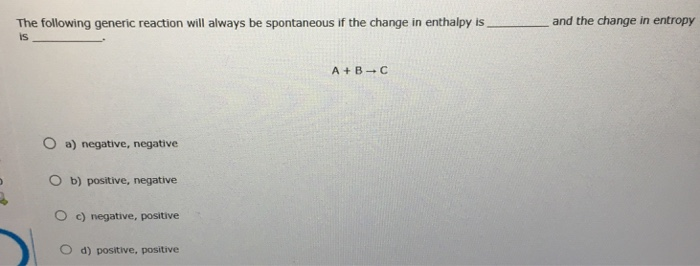# Problem: The following generic reaction will always be spontaneous if the change in enthalpy is________ and the change in entropy is _________. negative, negativepositive, negative negative, positive positive, positive

###### FREE Expert Solution

The equation of Gibbs Free Energy states that:

ΔG = ΔH-TΔS

93% (30 ratings)###### Problem Details

The following generic reaction will always be spontaneous if the change in enthalpy is________ and the change in entropy is _________.

negative, negative

positive, negative

negative, positive

positive, positive